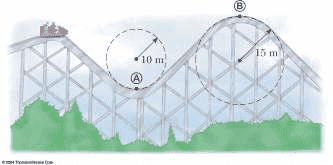# Force of a roller coaster track on a car

## Homework Statement

The roller coaster car shown below has a mass of 800 kg when fully loaded with passengers.
(a) If the car has a speed of 25.0 m/s at point A, what force does the track exert on the car at that
point. (b) If the car has a speed of 10.0 m/s at point B, what force does the track exert on the car.
(c) What is the maximum speed the car could have at B and still remain on the track?F = mv²/r

## The Attempt at a Solution

(a)
I thought I would just need to plug into the above formula but that didn't work. I did:
800kg * (25m/s)² / 10m and got the answer 50000N, but that is not the correct answer. I am not sure what else to
(b)
Edit: Figured out based on what I learned from part a.
(c)
Edit: Figured out based on what I learned from part a.

Last edited: